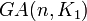# Isomorphic general affine groups implies isomorphic fields

## Statement

Suppose$K_1,K_2$ are fields and$n$ is a natural number. Suppose the general affine groups$GA(n,K_1)$ and$GA(n,K_2)$ are isomorphic groups. Then, the fields$K_1$ and$K_2$ are isomorphic fields.

## Proof

### Proof for the case$n = 1$

Step no. Assertion/construction Facts used Given data used Previous steps used Explanation
1 The derived subgroup is isomorphic to the additive group, and the quotient group (the abelianization) is isomorphic to the multiplicative group.
2 Choose any non-identity element of the derived subgroup as the field's "1" element. Then, we can identify the elements of the quotient with the elements of the derived subgroup based on where they send the element marked "1"
3 We thus have the entire field structure determined uniquely by the group structure.

### Proof for the case$n > 1$

In this case, the additive group of the vector space that's the base of the semidirect product is a minimal normal subgroup, hence is characteristic, and the quotient is isomorphic to the general linear group of degree$n$. We can then use the action of the center of this quotient group on this additive group by looking at the orbit of any element of that additive group under the center. This gives us a microcosm of a general affine group of degree one and we can use the proof for the case$n = 1$.